# Three Dimensional Geometry chapter 33 Terminal Exercise solutions NIOS

NIOS Mathematics 311 book 2 Module IX Chapter 36 check your progress 36.2 solutions are given.

You should study the textbook lesson Straight Line very well.

You should also observe and practice all problems and solutions given in the textbook.

Observe the given solutions and try them in your own method.

NIOS Maths 311 Book 2 solutions for some chapters

Three Dimensional Geometry

Terminal exercise chapter 33

Inter Three dimensional coordinates

## Maths 311 book 2 chapter 33 Terminal exercise solutions NIOS

Three Dimensional Geometry

Chapter 33

Terminal Exercise

1. Show that the points (0, 7, 10), (-6, 6, 6) and (-4, 9, 6) form an isosceles right-angled triangle.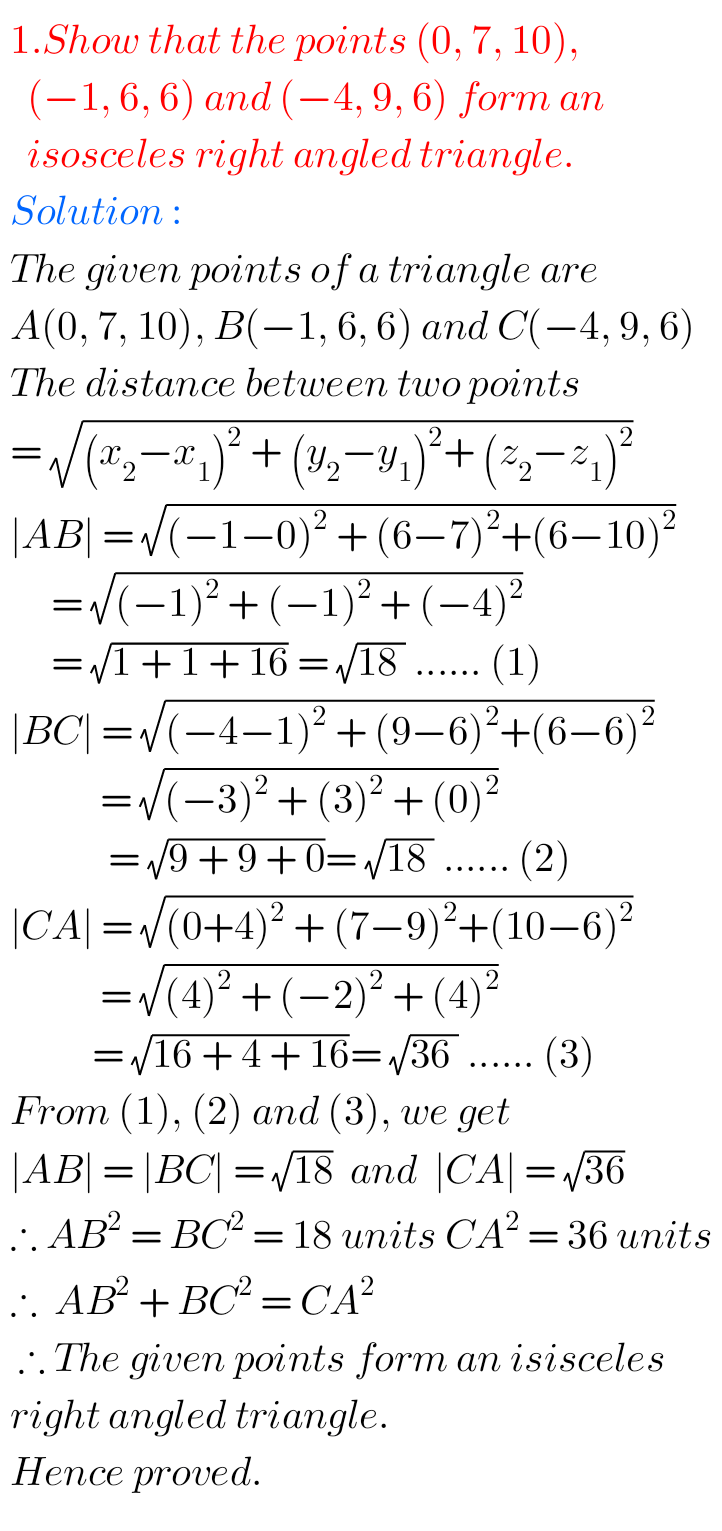2 Prove that the points, P, Q and R, whose coordinates are respectively (3, 2, -4), (5, 4, -6) and (9, 8, -10) are collinear and find the ratio in which Q divides PR.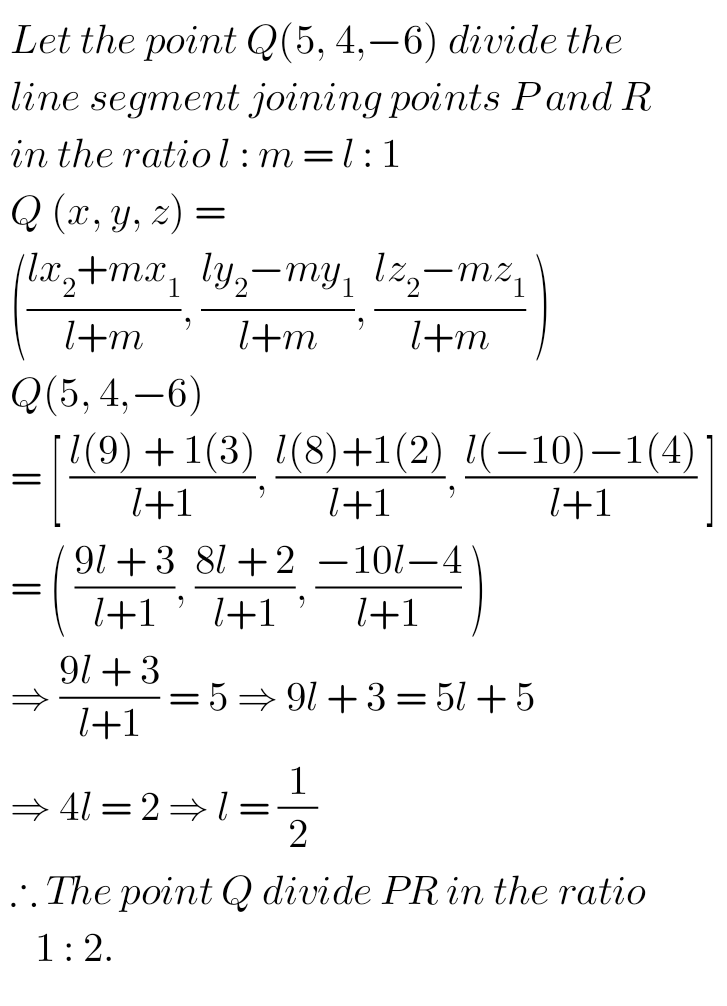3. Show that the points (0, 4, 1), (2, 3, -1), (4, 5, 0) and (2, 6, 2) are the vertices of a square.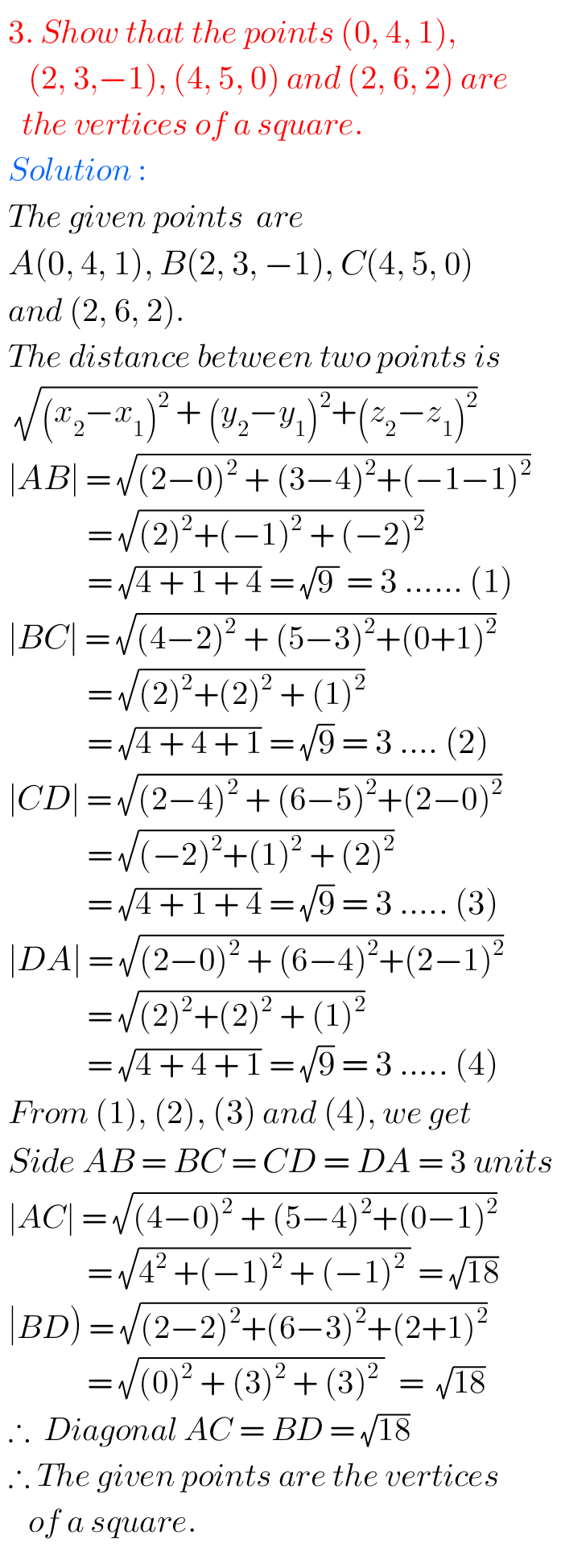### NIOS maths Three Dimensional Geometry Terminal Exercise solutions

4. Show that the points (4, 7, 8), (2, 3, 4), (-1, -2, 1) and (1, 2, 5) are the vertices of a parallelogram.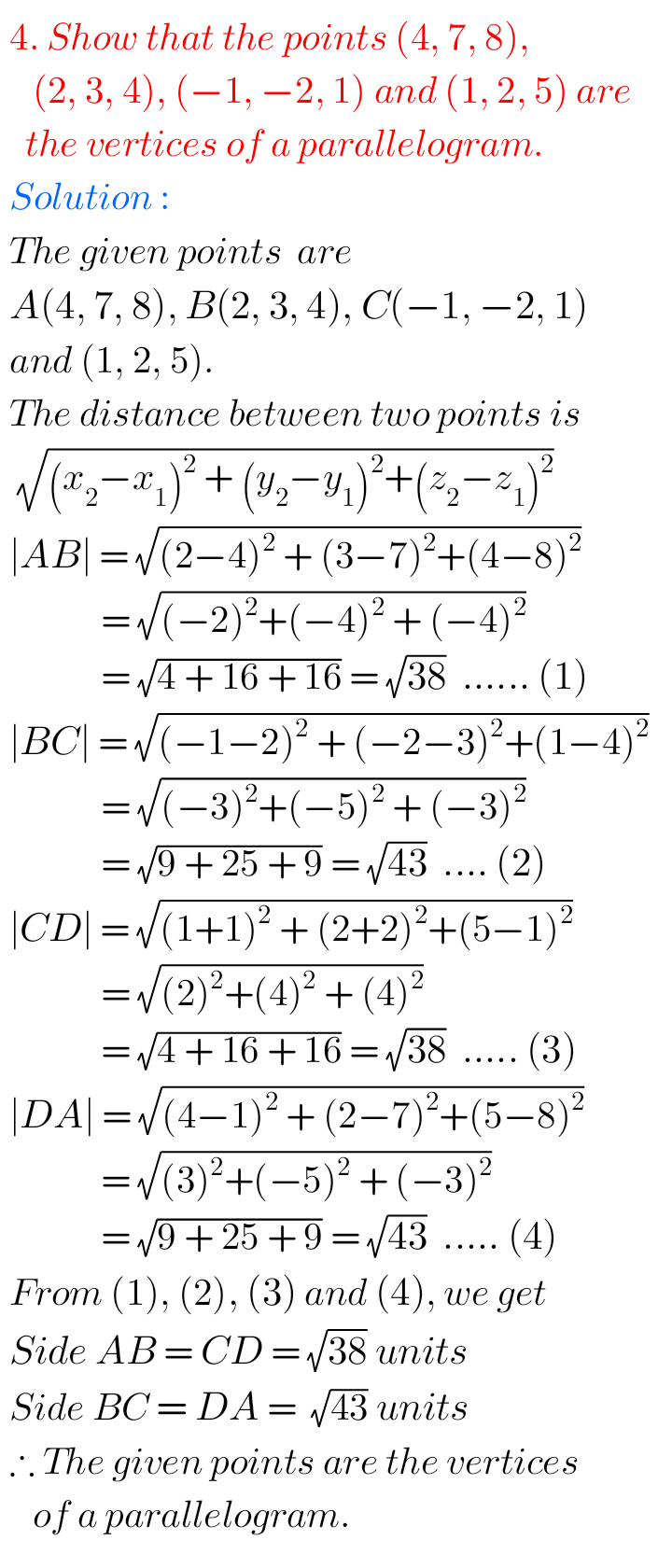5. Three vertices of a parallelogram ABCD are A (3, -4, 7), B (5, 3, -2) and C (1, 2, -3). Find the fourth vertex D.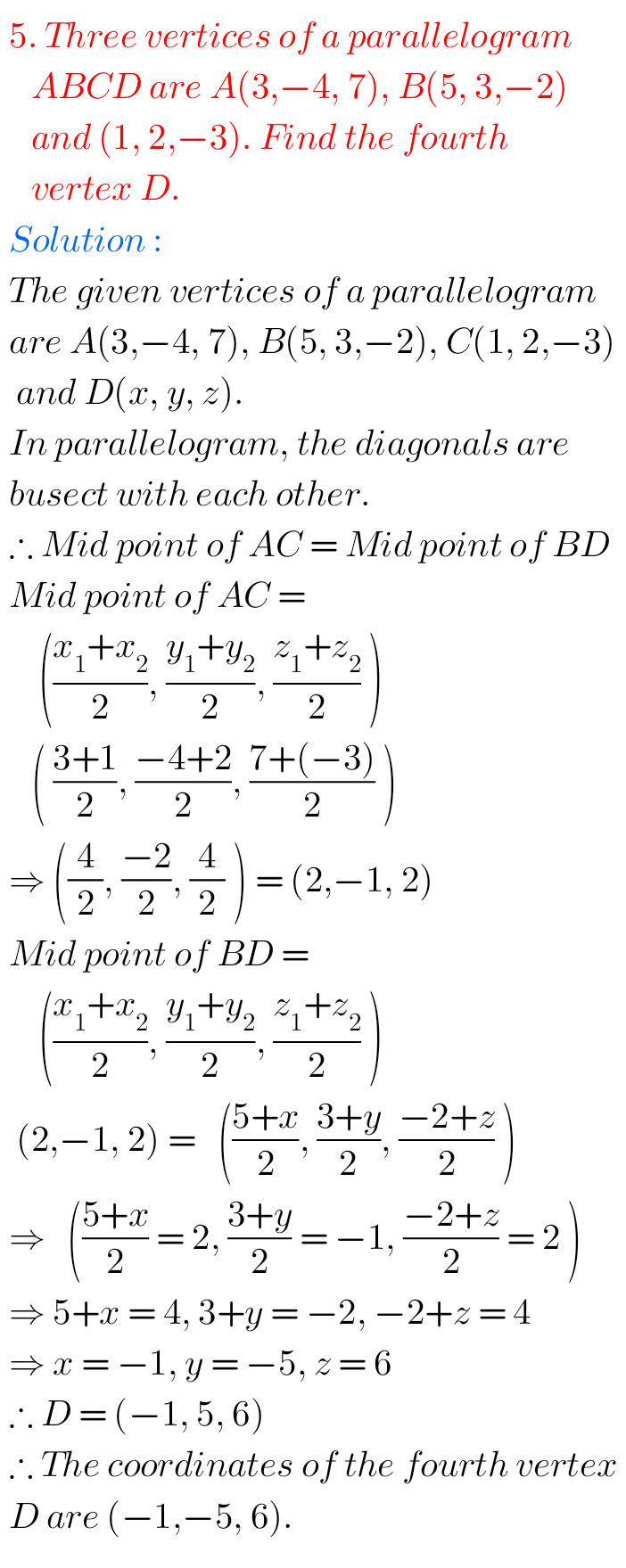Note: Observe the solutions and try them in your own method.

Inter maths solutions

SSC maths class 10 solutions

Ncert maths class 6 solutions